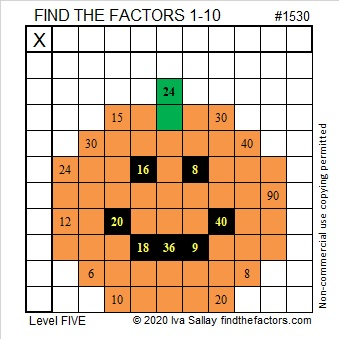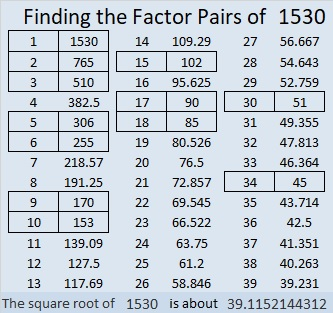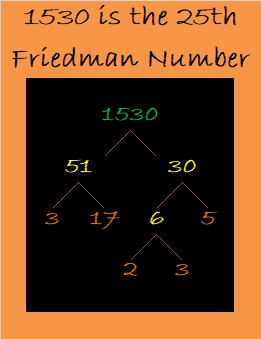# 1530 Jack-o’-lantern

Contents

### Today’s Puzzle:

Here’s a Jack-O’-Lantern Puzzle for you to enjoy. It’s a Level 5 puzzle so it might be more of a trick than a treat. Remember to use logic every step of the way instead of guessing and checking.Here’s the same puzzle without any added color:### Factors of 1530:

15 is half of 30, so 1530 is divisible by 6 just like all these numbers are divisible by 6: 12, 24, 36, 48, 510, 612, 714, 816, 918, 1020, 1122, 1224, 1326, 1428, and so forth.

• 1530 is a composite number.
• Prime factorization: 1530 = 2 × 3 × 3 × 5 × 17, which can be written 1530 = 2 × 3² × 5 × 17.
• 1530 has at least one exponent greater than 1 in its prime factorization so √1530 can be simplified. Taking the factor pair from the factor pair table below with the largest square number factor, we get √1530 = (√9)(√170) = 3√170.
• The exponents in the prime factorization are 1, 2, 1, and 1. Adding one to each exponent and multiplying we get (1 + 1)(2 + 1)(1 + 1)(1 + 1) = 2 × 3 × 2 × 2 = 24. Therefore 1530 has exactly 24 factors.
• The factors of 1530 are outlined with their factor pair partners in the graphic below.### More about the Number 1530:

51 × 30 = 1530. Did you notice that the same digits appear on both sides of the equal sign and only +, -, ×, ÷, (), or exponents were used to make a true statement? 1530 is only the 25th number that can make that claim, so we call it the 25th Friedman number.

There are MANY possible factor trees for 1530, but let’s celebrate that it is also a Friedman number with this one:1530 is the hypotenuse of FOUR Pythagorean triples:
234-1512-1530, which is 18 times (13-84-85),
648-1386-1530, which is 18 times (36-77-85),
720-1350-1530, which is (8-15-17) times 90, and
918-1224-1530, which is (3-4-5) times 306.

This site uses Akismet to reduce spam. Learn how your comment data is processed.